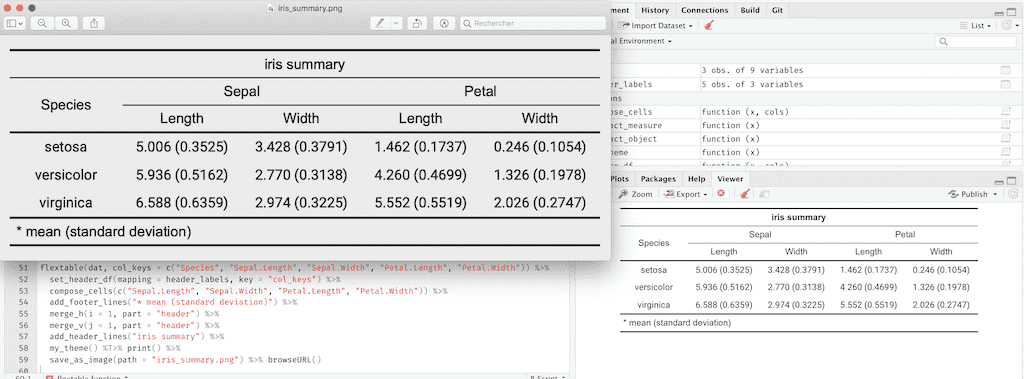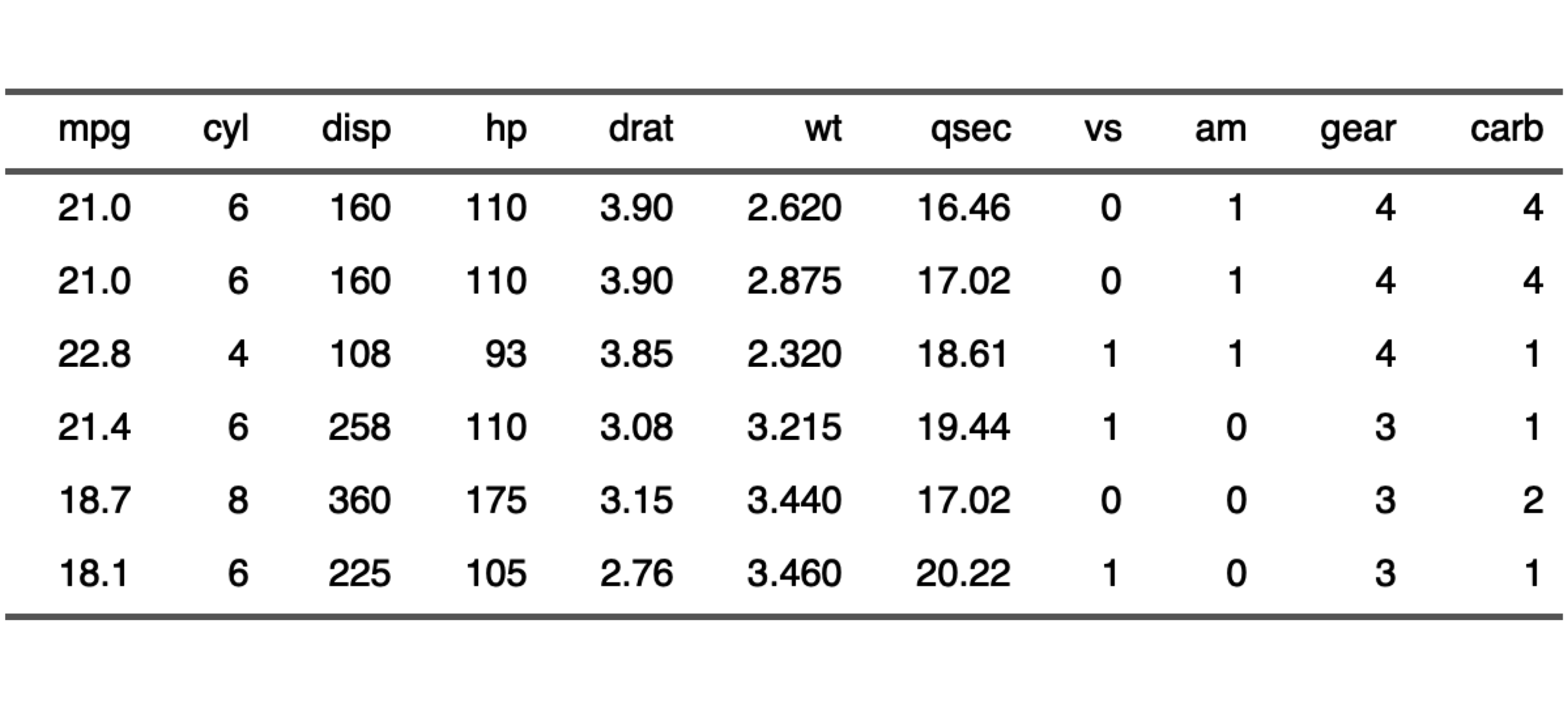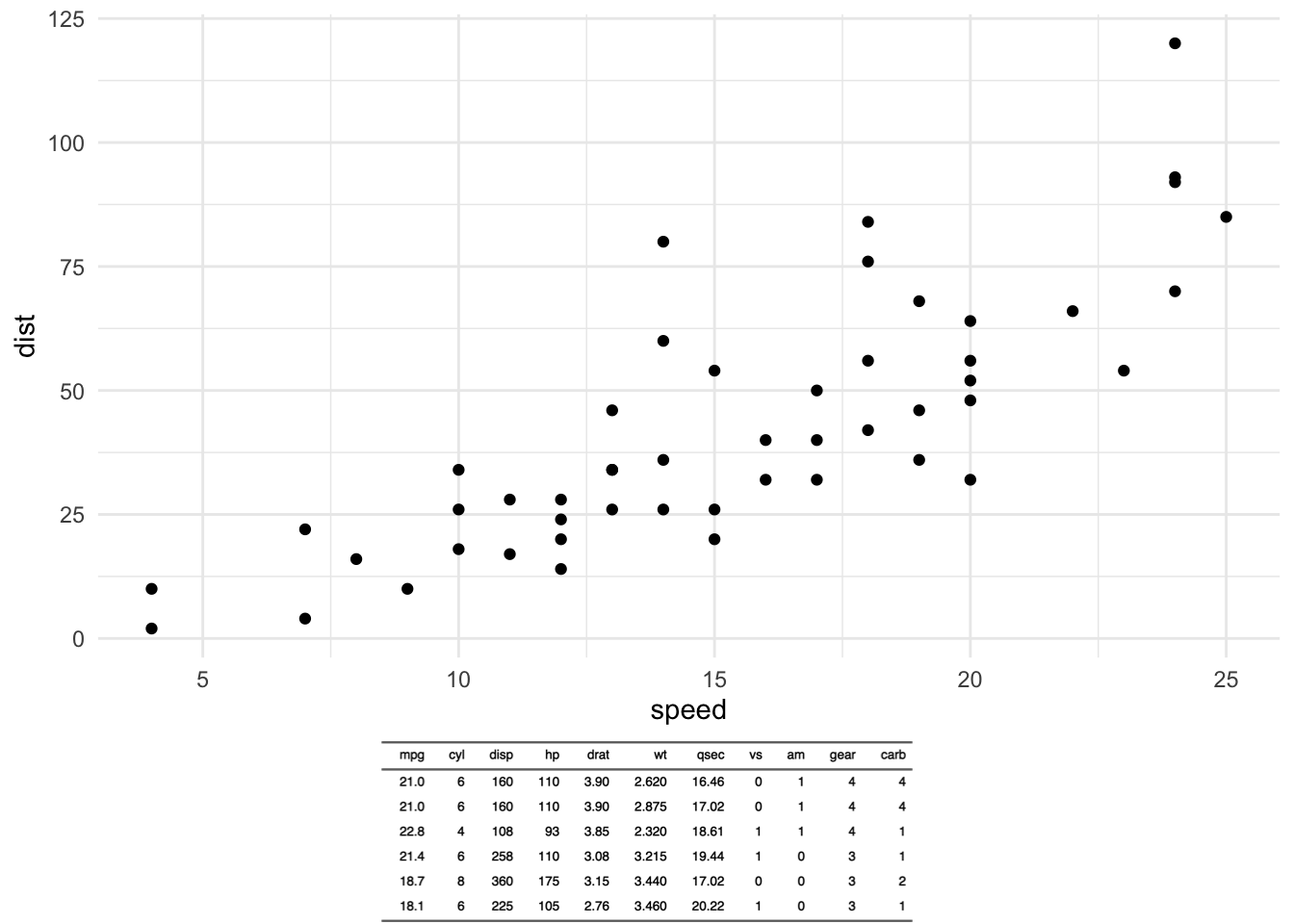# Save a flextable as an image

David Gohel

2019/05/23flextable 0.5.4 is now on CRAN. It contains a new output option that some users were asking: image output. You can now save a flextable as a png or pdf file with function save_as_image.

The solution was existing since a long time but was buried in a stackoverflow question instead of being provided in flextable as an option.

This functionality is letting other options to be available, you can now use method plot and also as_raster and do whatever you’d like with the raster (combine with a ggplot object for example).

## Demo

First, let’s create a simple flextable.

``````library(flextable)
ft <- flextable( head( mtcars ) )
ft <- autofit(ft)
ft``````
 mpg cyl disp hp drat wt qsec vs am gear carb 21.0 6 160 110 3.90 2.620 16.46 0 1 4 4 21.0 6 160 110 3.90 2.875 17.02 0 1 4 4 22.8 4 108 93 3.85 2.320 18.61 1 1 4 1 21.4 6 258 110 3.08 3.215 19.44 1 0 3 1 18.7 8 360 175 3.15 3.440 17.02 0 0 3 2 18.1 6 225 105 2.76 3.460 20.22 1 0 3 1

You can save it as a png:

``save_as_image(ft, path = "name.png")``

You can plot it:

``plot(ft)``Or combine the table with a ggplot object:

``````library(ggplot2)
library(grid)
library(cowplot)

ft_raster <- as_raster(ft)

anyplot <- qplot(speed, dist, data = cars, geom = "point")

gflextable <- ggplot() +
theme_void() +
annotation_custom(rasterGrob(ft_raster), xmin=-Inf, xmax=Inf, ymin=-Inf, ymax=Inf)

plot_grid(anyplot, gflextable, nrow = 2, ncol = 1, rel_heights = c(4, 1) )``````When a flextable is printed, it may be useful to know the exact width and height of the table to be sure to set up the correct aspect ratio. The function `flextable_dim()` will provide these informations without the need to produce the image.

``````dims <- flextable_dim(ft)
dims``````
``````#> \$widths
#>  6.212809
#>
#> \$heights
#>  2.035298
#>
#> \$aspect_ratio
#>  0.327597``````

You can reuse them as values for knitr chunk options `fig.asp`, `fig.width` and `fig.height`.